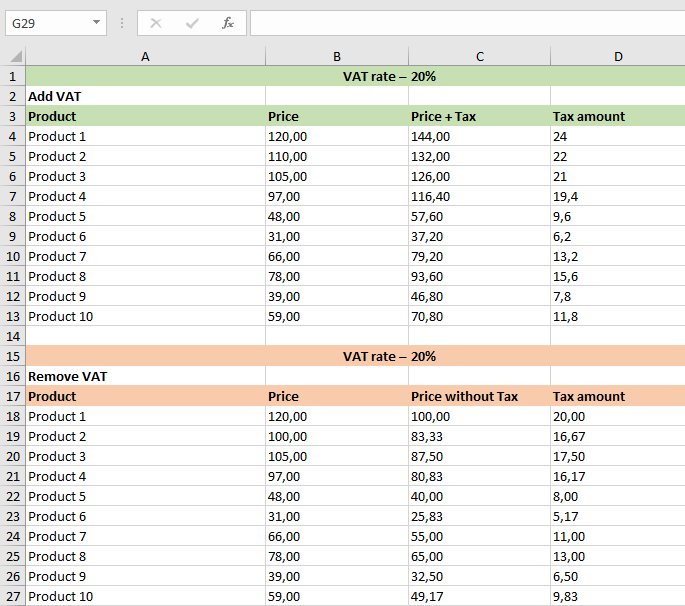# VAT Calculator Zimbabwe

Add or remove VAT in Zimbabwe in one click with our online VAT calculator. Just type the price inclusive or exclusive of VAT.
Thank you! Your submission has been received!
Oops! Something went wrong while submitting the form.
This is some text inside of a div block.
Thank you! Your submission has been received!
Oops! Something went wrong while submitting the form.

## How to work out VAT

Only 3 easy steps to calculate VAT onlineCheck the "VAT rate" (it’s pre-set to the UK standard rate of 20%)Enter the amount – net or gross price. Check full VAT rates list belowClick "Remove VAT" or "Add VAT" depending on what you want

## How to calculate VAT in math or Excel

Following these simple steps can help you to calculate VAT backwards or forwards.### Adding VAT formula

If you want to add VAT to the price, you just need to divide the price by 100 and then multiply by (100 + VAT rate). That's all, you got the price including VAT - Gross price.### Removing VAT formula

If you want to subtract VAT from the price, you need to divide the price by (100 + VAT rate) and then multiply by 100.  Now you know the price excluding VAT - Net price.### VAT calculation in Excel

If you need to calculate VAT for several products at once, it is easier to use Excel. I created a template – download it, it's free.Antony Kidless